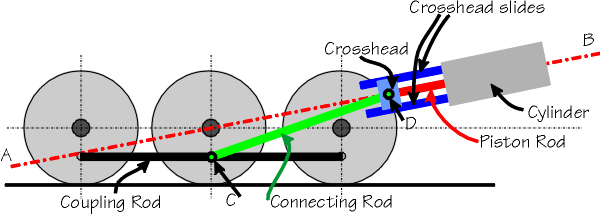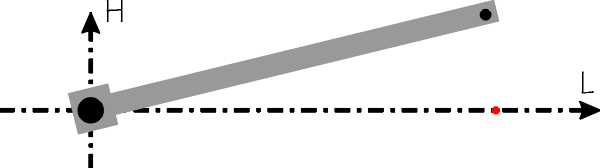# Rail3D*

 User Name: Password: Remember me next time.

# Steam Locomotive Valve Gear

Some notes on steam locomotive motion, with particular refernce to msts conversions

# Naming of parts

Just for reference, the important parts of the motion are labeled here: (See also http://home.new.rr.com/trumpetb/loco/)# Coupling Rod

The coupling rod couples the driving wheels together and is the simplest motion component to model. It is modeled using a simple motion1 keyword, eg

motion1 0/0/50 /CouplingRod

Motion1 is the same as an include statement, except that the sub-component is kept in a horizontal orientation, so if a motion1 component is connected to a wheel, it will move in a circular motion, but maintaining the horizontal orientation, and this is what we want for the coupling rod.

# Connecting Rod

The connecting rod is more complex and uses the motion2 keyword.

The connecting rod is mounted on the wheel at the same point as the coupling rod (point C in the above diagram). This point moves in a circular path around the wheel centre.

The other end of the connecting rod (D above) moves backwards and forwards along the cylinder centre line (line A/B above). Note that usually this line passes through the centre of the wheel the connecting rod is connected to.

The syntax for a Motion2 command is as follows:

motion2 w/l/h a/b/c ConnectingRod

w/l/h gives the mounting position on the parent component (C on the diagram), ie if the connecting rod is a sub-component of the driving wheel, w/l/h represent the position of point C relative to the wheel centre (this will often be the same as the w/l/h value of the motion1 component for the coupling rod). Thus w/l/h controls the motion of one end of the connecting rod, Point C in the above diagram.

a/b/c defines the motion of the other end of the connecting rod (D on the diagram above) as the wheel rotates. a is the distance between the two pivot points of the connecting rod, points C and D in the diagam. b/c defines the centre line of the cylinders (ab in the diagram), where c is the slope of the line and b is the vertical offset of the line.

## Setting up a connecting rod.

Assuming you have already set up the wheels and the coupling rod, there is a relativly easy step by step process of setting up the conn rod. Before starting work on the conn rod, check:

• The wheels are set up and rotating properly
• The coupling rod has been added with a Motion1 keyword and moves correctly
• The piston and/or crosshead slides are modelled (as you need something to check the motion against)

Step by step

1. Measure the length (Distance C/D on the diagram) of the connecting rod (preferably from a drawing)
2. Create a “Working component” for the connecting rod, consisting of a single panel of the same length as the connecting rod: fill 0/0/−5 0/0/5 0//5 0//−5
3. Add this working component to the model with a Motion2 keyword, set the w/l/h so that the base of the component rotates around the wheel, set the remaining terms to the length, slope 0 and offset 0:/0/0
4. Switch on animation in the editor and check the movement of the new con rod: End C should move around the wheel as the wheel rotates, the other end should move horizontally along the ground.
5. Measure the slope of the cylinder centre line (ab on the diagram) from the loco drawing and add this to your motion2 statement: Motion2 w/l/h/0/
6. You should now have end D of the working component moving along the specified slope, it probably won’t be on the cylinder centre line, but it should be moving parallel to the cylinder centre line. Depending on the orientation of your model, you may need to use a negative value for the slope. Adjust the slope term until the D end of the conn rod is moving parallel to the cylinder center line A/B
7. Once the D end is moving parallel to the cylinder centre line, adjust the vertical term to move end D up or down until it on the line ab
8. Once you have got the simple single fill conn rod moving correctly, substitute your more detailed model, or develop the single fill as necessary.

Further points:

• Setting up the motion with simple single fill components is recommended to understand the motion terms: detail can be added later.
• I could produce a spreadsheet to calculate the slope and offset values, but to be honest I find the above step by step process easy enough and tend to use that.

# Piston Rod

The piston rod and crosshead assembly are easy once the connecting rod is set up properly.

The piston rod will be mounted on the connecting rod (at end D), and will have the same slope and offset terms as the conn rod since it moves along the same line A/B. The length term of the motion2 (fourth term) should be set to the length of the piston rod, although it is not critical in this case.

# Motion components in msts conversions

When converting an msts model, it is recommended that the motion is set up with simple single fill components as above. This allows you to get the motion set up properly before worrying about the detail and appearance of the components.

## Important note

The rail3d motion keywords are designed for components aligned along the L axis:and the maths used to calculate the position of the component aligns the L axis of the sub-component.

Unfortunatly, this is often not the case for msts conversions, thus a connecting rod converted from msts is often not aligned to the L axis:If this is the case, it is difficult to calculate the slope and offset terms for the Motion2 statement.

I also suspect (hopefully someone better at maths can confirm this) that it is not possible to correctly animate such a component with the Motion2 command: since the motion 2 command moves the point on the L axis (shown by the red mark above) along the straight line A/B, the end of the conn rod offset as above will not move in a straight line.

Solutions to this problem:

• Modify the original msts model (where possible) so that the conn rod component (and piston rod) is aligned along the L axis.
• Discard the converted component and create a new with the correct alignment (you could still use the textures from the msts conversion).

MRG 05/06/2013 12:14:10